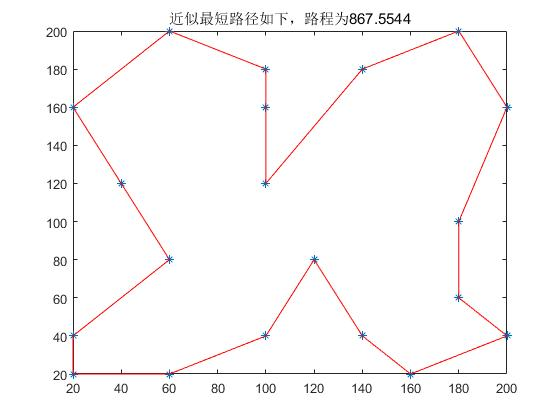### 引言

模拟退火算法基于Monte-Carlo迭代求解策略的一种随机寻优算法，其出发点是基于物理中固体物质的退火过程与一般组合优化问题之间的相似性。模拟退火算法从某一较高初温出发，伴随温度参数的不断下降,结合概率突跳特性在解空间中随机寻找目标函数的全局最优解，即在局部最优解能概率性地跳出并最终趋于全局最优。模拟退火算法是一种通用的优化算法，理论上算法具有概率的全局优化性能,目前已在工程中得到了广泛应用，诸如VLSI、生产调度、控制工程、机器学习、神经网络、信号处理等领域。

模拟退火算法是通过赋予搜索过程一种时变且最终趋于零的概率突跳性，从而可有效避免陷入局部极小并最终趋于全局最优的串行结构的优化算法。

• 从一个问题来看看：

假定有一个定义域 $x$，需要求函数$f(x)$在$X$上的最优解，这里以取最小值为例（取最大值类似）

1. 如果 $X$ 离散且取的是有限值，那么通过穷取就能够得到最优解；
2. 如果 $X$ 连续，且函数$f(x)$是一个凹函数，那么通过梯度下降，三分法等来求得最小值；
3. 但是如果函数连续且非凹，就有可能只是求得局部最优解的情况；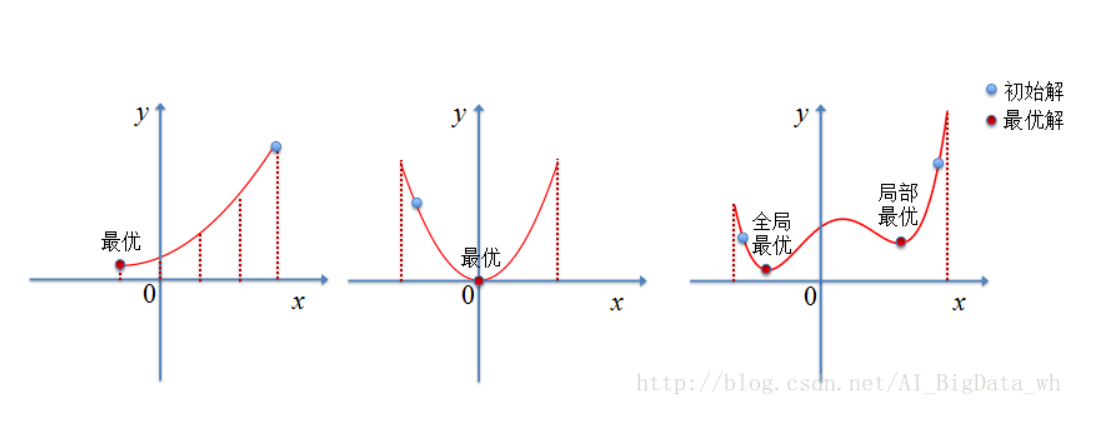### 原理

根据Metropolis准则，粒子在温度T时趋于平衡的概率为$e(-ΔE/(kT))$，其中E为温度T时的内能，ΔE为其改变量，k为Boltzmann常数。用固体退火模拟组合优化问题，将内能E模拟目标函数值f，温度T演化成控制参数t，即得到解组合优化问题的模拟退火算法：由初始解i和控制参数初值t开始，对当前解重复“产生新解→计算目标函数差→接受或舍弃”的迭代，并逐步衰减t值，算法终止时的当前解即为所得近似最优解，这是基于蒙特卡罗迭代求解法的一种启发式随机搜索过程。退火过程由冷却进度表(Cooling Schedule)控制，包括控制参数的初值t及其衰减因子Δt、每个t值时的迭代次数L和停止条件S。

### 基本思想

模拟退火其实也是一种贪心算法，但是它的搜索过程引入了随机因素。在迭代更新可行解时，以一定的概率来接受一个比当前解要差的解，因此有可能会跳出这个局部的最优解，达到全局的最优解。以下图为例，假定初始解为左边蓝色点A，模拟退火算法会快速搜索到局部最优解B，但在搜索到局部最优解后，不是就此结束，而是会以一定的概率接受到左边的移动。也许经过几次这样的不是局部最优的移动后会到达全局最优点D，于是就跳出了局部最小值。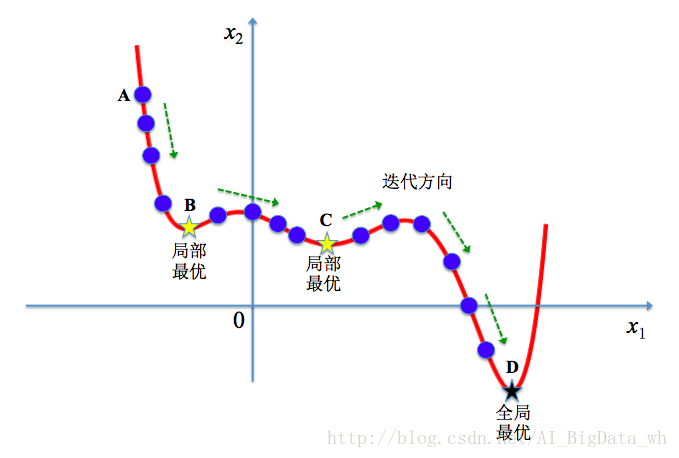根据Metropolis准则，粒子在温度T时趋于平衡的概率为 $exp(- \frac{ΔE}{kT})$，其中E为温度T时的内能，$ΔE$为其改变数,$k$为Boltzmann常数。Metropolis准则常表示为：Metropolis准则表明，在温度为$T$时，出现能量差为$dE$的降温的概率为$P(dE)$，表示为：
$$P(dE) = exp( \frac{dE}{kT} )。$$

我们将一次向较差解的移动看做一次温度跳变过程，我们以概率P(dE)来接受这样的移动。也就是说，在用固体退火模拟组合优化问题，将内能E模拟为目标函数值 f，温度T演化成控制参数 t，即得到解组合优化问题的模拟退火演算法：由初始解 i 和控制参数初值 t 开始，对当前解重复“产生新解→计算目标函数差→接受或丢弃”的迭代，并逐步衰减 t 值，算法终止时的当前解即为所得近似最优解，这是基于蒙特卡罗迭代求解法的一种启发式随机搜索过程。退火过程由冷却进度表(Cooling Schedule)控制，包括控制参数的初值 t 及其衰减因子Δt 、每个 t 值时的迭代次数L和停止条件S。

• 若$f( Y(i+1) ) <= f( Y(i) )$ (即移动后得到更优解)，则总是接受该移动；
• 若 $f( Y(i+1) ) > f( Y(i))$ (即移动后的解比当前解要差)，则以一定的概率接受移动，而且这个概率随着时间推移逐渐降低（逐渐降低才能趋向稳定）相当于上图中，从B移向BC之间的小波峰时，每次右移（即接受一个更糟糕值）的概率在逐渐降低。如果这个坡特别长，那么很有可能最终我们并不会翻过这个坡。如果它不太长，这很有可能会翻过它，这取决于衰减 t 值的设定。

• 普通Greedy算法：兔子朝着比现在低的地方跳去。它找到了不远处的最低的山谷。但是这座山谷不一定最低的。这就是普通Greedy算法，它不能保证局部最优值就是全局最优值。
• 模拟退火：兔子喝醉了。它随机地跳了很长时间。这期间，它可能走向低处，也可能踏入平地。但是，它渐渐清醒了并朝最低的方向跳去。这就是模拟退火。

### 描述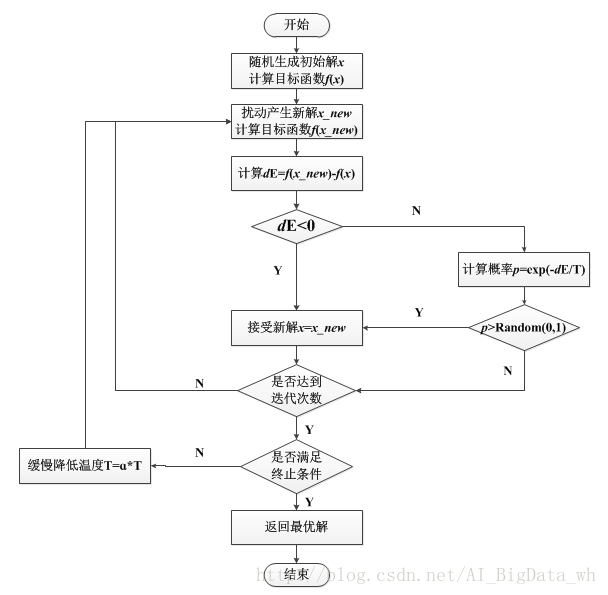### 步骤

• 第一步：

由一个产生函数从当前解产生一个位于解空间的新解；为便于后续的计算和接受，减少算法耗时，通常选择由当前新解经过简单地变换即可产生新解的方法，如对构成新解的全部或部分元素进行置换、互换等，注意到产生新解的变换方法决定了当前新解的邻域结构，因而对冷却进度表的选取有一定的影响。

• 第二步：

计算与新解所对应的目标函数差。因为目标函数差仅由变换部分产生，所以目标函数差的计算最好按增量计算。事实表明，对大多数应用而言，这是计算目标函数差的最快方法。

• 第三步：

判断新解是否被接受,判断的依据是一个接受准则，最常用的接受准则是Metropolis准则:ΔT<0则接受S′作为新的当前解S，否则以概率exp(-ΔT/T)接受S′作为新的当前解S

• 第四步：

当新解被确定接受时，用新解代替当前解，这只需将当前解中对应于产生新解时的变换部分予以实现，同时修正目标函数值即可。此时，当前解实现了一次迭代。可在此基础上开始下一轮试验。而当新解被判定为舍弃时，则在原当前解的基础上继续下一轮试验。

### 缺点

1. 温度T的初始值设置问题

温度TT的初始值设置是影响模拟退火算法全局搜索性能的重要因素之一、初始温度高，则搜索到全局最优解的可能性大，但因此要花费大量的计算时间；反之，则可节约计算时间，但全局搜索性能可能受到影响。

2. 退火速度问题，即每个TT值的迭代次数
模拟退火算法的全局搜索性能也与退火速度密切相关。一般来说，同一温度下的“充分”搜索是相当必要的，但这也需要计算时间。循环次数增加必定带来计算开销的增大。

3. 温度管理问题
温度管理问题也是模拟退火算法难以处理的问题之一。实际应用中，由于必须考虑计算复杂度的切实可行性等问题，常采用如下所示的降温方式：

$$T= \alpha \times T \alpha \in(0,1)$$

### 实例

#### 实例1

$$f(x)=(x-2) \times (x+3) \times (x+8) \times(x-9)$$

python程序求解如下：

#### 实例2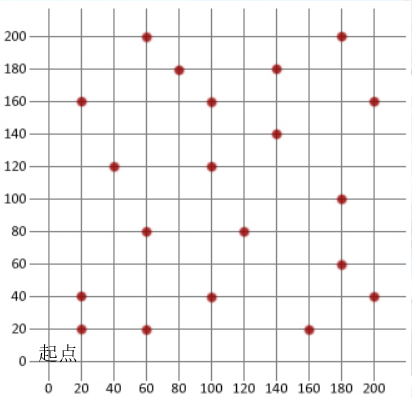$$d_{AB}= \sqrt{({x_A-x_B})^2+({y_A-y_b})^2}$$

1. 解空间：从W1（标号为1）出发，目的地一次编号为2，3，·····，20，最后再回到W1（编号为21），解空间S可以描述为{1，2，3，······，21}的所有固定起点和终点的循环排列集合，即：

$$S={C_1,···,C_{21} | C_1=1,(C_2,···,C_{20})为{2，3，···,20}的排列循环，C_{21}=21}$$

​ 每一个循环排列表示19个目标的一个回路，$C_i=j$为第$i-1$次到达目的地$j$，初始解可以选择（1，2，3，···，21），也可以先使用蒙特卡洛方法求得一个较好的初始解

1. 目标函数。目标函数（或称之为代价函数）为侦察所有目标的路径长度。要求
$$min f(C_1,···,C_{21})=\sum_{i=1}^{21}d_{c_ic_{i+1}}$$
而一次迭代由下列三步构成

2. 新解的产生。设上一步迭代的解为：
$$C_1···C_{u-1}C_uC_{u+1}···C_{v-1}C_vC_{v+1}···C_{w-1}C_wC_{w+1}···C_{21}$$

• 2变换法。任选序号u，v，交换二者的顺序,此时的新路径为：
$$C_1···C_{u-1}C_vC_{v+1}···C_{u+1}C_uC_{v+1}···C_{21}$$

• 3变换法。任选序号u，v，w，将u，v之间的路径查到w之后，对应的新路径为：
$$C_1···C_{u-1}C_{v+1}···C_{w}C_u···C_{v}C_{w+1}···C_{21}$$

3. 代价函数差。对于2变换法，路径差可表示为
$$\Delta f=(d_{c_{u-1}c_v}+d_{c_{u}c_{v+1}})-(d_{c_{u-1}c_u}+d_{c_{v}c_{v+1}})$$

4. 接受准则如果$\Delta f<0$，则接受新的路径；否则，以概率$exp(- \Delta f/T)$接受新的路径，即用计算机产生一个[0,1]区间上均匀分布的随机数rand，若$rand \le exp(- \Delta f/T)$则接受

5. 降温。利用选定的降温系数 α 进行降温，取新的温度 T 为 αT（这里 T 为上一步迭代的温度），在此选定 α =0.999

6. 结束条件。用选定的终止温度 $e = 10^{-30}$，判断退火过程是否结束。若T<e，则算法结束，输出当前状态

Matlab代码